Drive_Index
Gears Gearboxes

Bevel Gears

Introduction

There are four basic types of bevel gears.

 Straight bevel gears.. These gears have a conical pitch surface and straight teeth tapering towards an apex Zerol bevel gears. These are similar to a bevel gears except the teeth are curved.   In essence, Zerol Bevel Gears are Spiral Bevel Gears with a spiral angle of zero. Spiral bevel gears. The teeth are curved teeth at an angle allowing tooth contact to be gradual and smooth Hypoid bevel gears..These gears are similar to spiral bevel except that the pitch surfaces are hyperboloids rather than cones.   Pinion can be offset above, or below,the gear centre, thus allowing larger pinion diameter, and longer life and smoother mesh, with additional ratios e.g 6:1, 8:1, 10:1

Specifications

BS 545:1982..Specification for bevel gears (machine cut)..(Obsolescent)
BS ISO 10300-1:2001..Calculation of load capacity of bevel gears. Calculation of load capacity of bevel gears. Introduction and general influence factors
BS ISO 10300-2:2001..Calculation of load capacity of bevel gears. Calculation of surface durability (pitting)
BS ISO 10300-3:2001..Calculation of load capacity of bevel gears. Calculation of tooth root strength

Straight bevel gears

These are gears cut from conical blanks and connect intersecting shaft axes.  The connecting shafts are generally at 90o and sometimes one shaft drives a bevel gear which is mounted on a through shaft resulting in two output shafts.    The point of intersection of the shafts is called the apex and the teeth of the two gears converge at the apex.   The design of bevel gears results in thrust forces away from the apex.   With the bearing limitations the gears have to be carefully designed to ensure that they are not thrown out of alignment as they are loaded.

Straight bevel gears are used widely in machine drive systems to effect 90o direction changes. and in differential drives    They have the same limitations as spur gears and they are therefore not used on high duty high speed applications.  Straight bevel gears are low cost units supplied with ratios from 1:2 to 4:1.

Straight Bevel Gear Terminology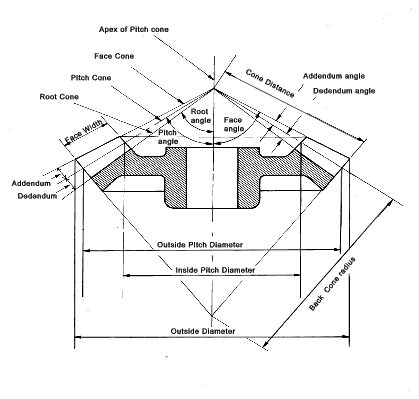Straight Bevel Gear Force equations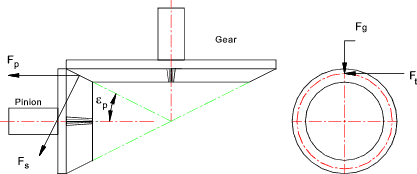Nomenclature

z p = Number of teeth on pinion
z g = Number of teeth on pinion
α = Pressure Angle of Teeth.
ε p = Pitch Angle (pinion)....= tan-1 (z p / z g )
ε g = Pitch Angle (gear)....= tan-1 (z g / z p )
P p = Power at Pinion shaft (kW)
n p = Rotational speed of pinion shaft (revs/min)
d p = Pinion Pitch Circle diameter (mm)
Mp = torque on pinion shaft (Nm)
Fs = Separating Force (N)
Fp = Pinion Thrust (N)
Fg = Gear Thrust (N)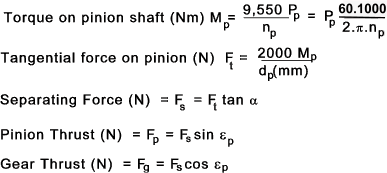Zerol Bevel Gears

The advantage of Zerol bevel gears compared to straight bevel gears is that they operate with a smooth localised point contact as opposed to a line contact enabling smoother operation with low vibration levels and higher speeds.  Because there is no spiral angle and no additional developed thrust these gears can be used as direct replacements for straight bevel gears.   These gears normally have a pressure angle of 20 o.  The minimum number of teeth on the pinion is 14.  The design of Zerol gears is relatively specialised and they are manufactured using special "Gleason" machine tools..

Spiral Bevel Gear

These are produced using a spiral gear form which results in a smoother drive suitable for higher speed higher loaded applications.  Again satisfactory performance of this type of gear is largely dependent upon the rigidity of the bearings and mountings.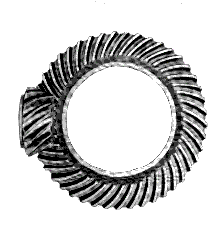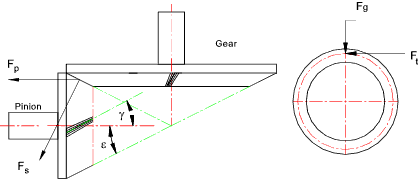α n = Normal Pressure angle..
ε = pitch cone angle
γ = Helix angle

Pinion Thrust F p = F t [ (tan α n sin ε / cos γ ) ± tan g cos ε ]

Note: ( + ) if helix angle is as shown and ( -) if helix angle is opposite to that shown

Hypoid Bevel Gear

Hypoid gears are best for the applications requiring large speed reductions with non intersecting shafts and those applications requiring smooth and quiet operation.  Hypoid gears are generally used for automotive applications.  The minimum number of teeth for speed ratios greater than 6 :1 is eight although 6 teeth pinions can be used for ratios below 6:1.   Hypoid gears have pressure angles between 19 and 22o.    The design of hypoid gears is relatively specialised and they are manufactured using special "Gleason" machine tools..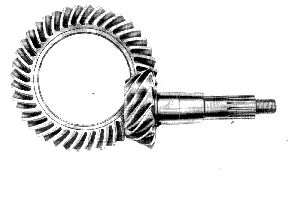Straight Bevel Gear Strength and Durability Equations

Designing bevel gears is normally done in accordance with standards as listed under specifications above: The notes below relate to approximate methods for estimating gear strengths.   The methods are really only useful for first approximations and/or selection of stock gears (ref links below). — Detailed design of bevel gears should only be completed using the relevant standards.   Books are available providing the necessary guidance.   Software is also available making the process very easy.

The equations are basically modified spur gears equations using a spur gear equivalent number of teeth z e

Equivalent Number of teeth on gear = z eg = z g / cos ε g
Equivalent Number of teeth on pinion = z ep = z p / cos ε p

Bending Strength Equations

The basic lewis formula for spur gear teeth is shown as follows

s = F t / ( W. m. Y )

 F t = Tangential force on tooth (N) s = Tooth Bending stress (MPa) W = Face width (mm) Y = Lewis Form Factor m = Module (mm)

The Lewis formula is modified to provide the allowable tangential force F b based on the allowable bending Stress S b

F a = S b.W. m. Y

It is clear that a bevel gear does not have a uniform section or a uniform module and therefore it is necessary to start an analysis by considering an element dx..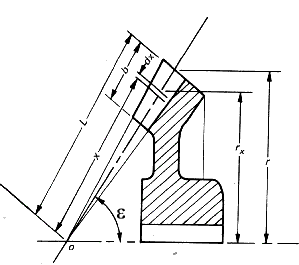The Lewis formula applied to the element is as follows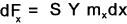To obtain the allowable torque (M) transmitted by the gears multiply both sides by r xand integrate the resulting equations as shown below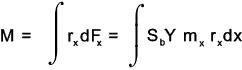The module varies along the gear teeth in proportion to the radius from the apex along the pitch cone.
Thus ..m / m x = L / x where m = module at x = L

A similar relationship holds for for r x. i.e for r x /r = x /L
Substituting these relationships into the integration equation results..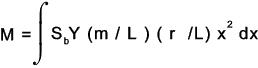d x varies from x = (L -b) to x = L the integration can be solved as follows: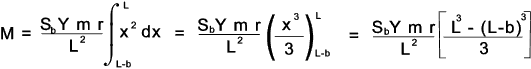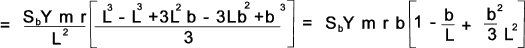The face width is considered to be limited to 1/3 of the cone distance when the factor b2 / (3.L2) = 1/27 is so small compared to the other factors that it can be reasonably ignored .    Then dividing by r to arrive at the Lewis equation for the allowable bending load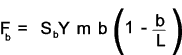The allowable bending load F b must be greater than the dynamic load which is the actual bending load calculated from the transmitted torque modified by the Barth formula as identified in the notes on spur gears i.e

F b ³ F t / K v

K v is given by the Barth equation for milled profile gears.

K v = 6,1 / (6,1 +V )

Note: This factor is different for different gear conditions i.e K v = ( 3.05 + V )/3.05 for cast iron, cast profile gears.

V =Average velocity of gear face = 0.0000524.n.d mean
d mean is the mean pitch circle diameter (mm)..
n = Rotational speed of gear (rpm)

Surface Durability Equations

The gear durability equation is based on the Hertz contact stress equation and its application to gears.
The allowable tangential wear load F w is calculated as follows

F w = d p. K. Q' / cos ε p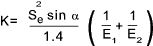d p = Pitch diameter measured at the back of tooth
Q' = 2 z eg /( z ep + z ep )
z eg & z ep are the equivalent number of teeth on the gear and pinion as defined above
K = Wear Load Factor see table Gear table

The allowable load F w must be greater than the dynamic bending load which is the actual load calculated from the transmitted torque modified by the Barth formula as identified in the notes on spur gears i.e

F w ³ F t / K v

 Links to Bevel Gears Boston Gears - Miter Gears ...A supplier of bevel gears NASA TEsts on Spiral Bevel Gears ...Interesting short note. Neeterdrive.co.uk ... UK supplier of Bevel gears and associated components SEW Eurodrive...All the information on Gearboxes you will need Quality Transmission Components...Supplier with downloadable Gear Design Handbook Stock Drive Products= Sterling Instruments...Supplier with large quantity of downloadable drive information hewitt-topham...UK gear supplier Lenze...Drive system supplier with geared motor section